I.7.1

9/16/2021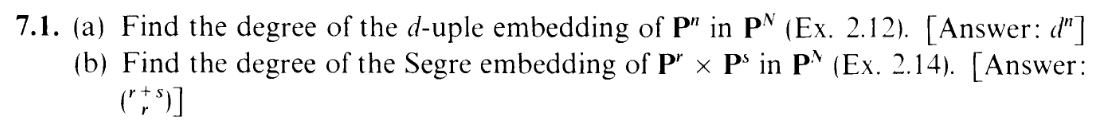Welcome to the new section, reader! Spread your arms! Let's do math! First off, here's a spam of definitions n stuff: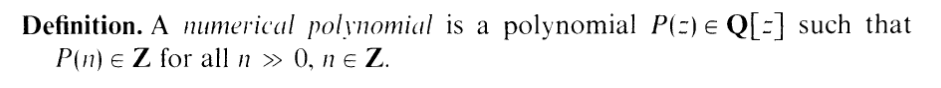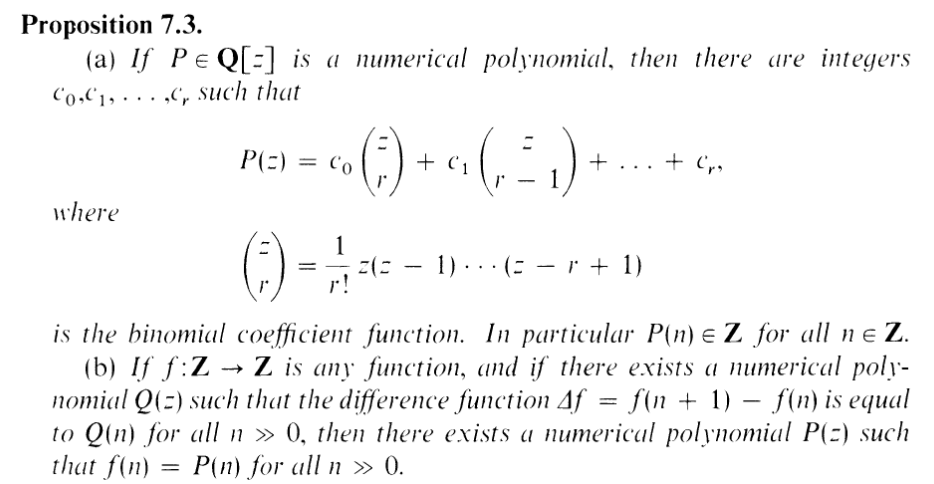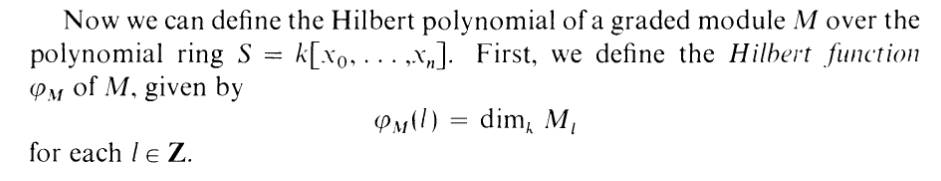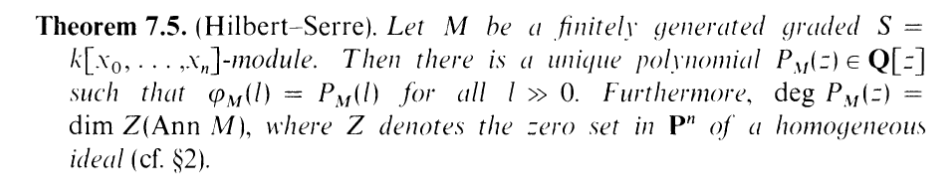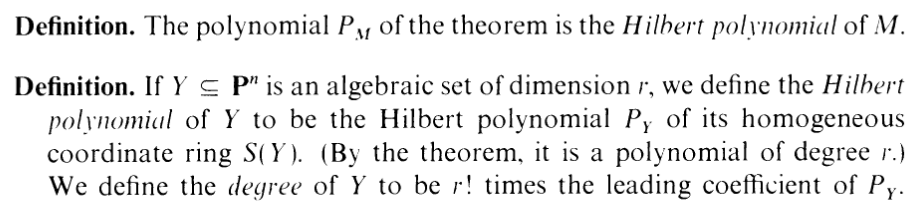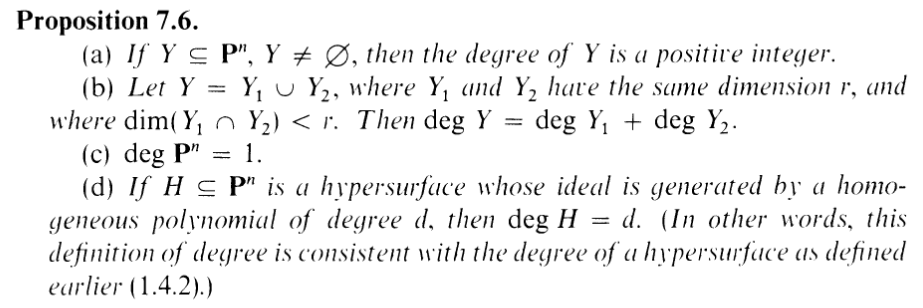whew. Yea, I actually read most of that, including the proofs (..most of it.). ANYWAY... part A wants us to calculate the degree of the d-uple embedding from 2.12 (holy shit that exercise was a lot of work lol).

ALSO: Hartshorne is generous enough to give us the answer: dn. So our job is to prove it.

Here's an interesting thing tho: degree is NOT preserved over isomorphism. If you look at Prop 7.6(c) above, you'll see that deg Pn = 1. But Pn is isomorphic to its d-uple embedding in PN (2.12c), YET, the degree of the embedding is certainly not 1. Kinda weird to talk about stuff that isn't isomorphism independent, tbh, but w.e. i guess well roll w it.

OK: so let's bring back some of the notation from 2.12. The d-uple embedding of Pn into PN is given by Z(α), where α = ker θ, and θ : k[y0,,yN] k[x0,,xn] sends the yis to d-degree monomials over x0,,xn. Hence, to calculate the degree of Y = Z(α), we want to calculate the Hilbert polynomial of S(Y ) = k[y0,,yN]∕α, and multiply the leading coefficient by n! (the d-uple embedding of Pn is isomorphic to Pn, hence the dimension is n)...... except we don't. Not quite. ysee, I struggled my balls off trying to calculate the Hilbert polynomial of S(Y ) as given above.... and so I looked at the solution. SORRY. Here's the trick: use the
isomorphism theorem, and realize that S(Y ) imθ.... Yea... it's WAY easier to analyze the image of θ in k[x0,,xn], lol. Use the image, not the quotient. Got it. let's do that.

BTW: I'm inherting the grading of M = imθ via the isomorphism. So M1 is all the d-degree elements in k[x0,,xn], M2 are all the 2d-degree elements, etc. Then, obviously, Ml is generated by the l-degree monomials over the indeterminates x0,,xn. So we need to COUNT (up to constant multiple). Get in here combinatorics fellas. How many ld degree monomials can you make out of n + 1 indeterminates. FOLKS: from x0,,xn, you need to pick ld of them. Order doesn't matter, and you can reuse elements. So it must be.... a COMBINATION WITH REPLACEMENT. I.e. the one I have to look up the formula for. HENCE, we can write the Hilbert function (polynomial) as

 ϕ(l) =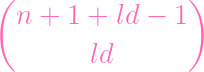=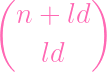=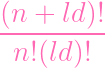=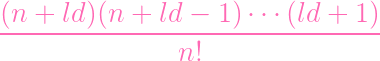As per the definition of degree, let's multiply by n!, yielding just the numerator

 (n + ld)(n + ld - 1)(ld + 1)

Of course, we care about the leading coefficient of this term (seen as a polynomial over l). And would you look at that? There's exactly n terms here, so the leading term is dnln, and the leading coefficient is dn. DONE. NOTE THAT n here is NOT the dimension of the ambient space of the embedding. That is N. n is the dimension of the preimage of the embedding. i know this is bad gibberish but it makes sense to me lol

### BOkay, same deal, except this time we care about the thing from 2.14:

 θ : k[{zij] → k[x0,…,xr,y0,…,ys] zij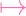xiyj

OK: Same trick as last time (USE THE IMAGE, NOT THE QUOTIENT). Let's look at M = imθ instead of the quotient. GOT IT? Alright. And this time the grading is: M1 is generated by the 2-degree monomials equally split between x, y. M2 is generated by the 4-degree monomials equally split between x, y. Etc. COUNTING TIME AGAIN. To count all the monomials (up to constant multiple) in Ml, we're combining l elements from x0,,xr (with replacement), then combining l elements from y0,,xs (with replacement). To pick the xs, we use the same formula from part A:=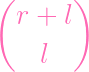Similarly, to pick the ys, it's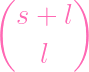So, using the rule of product (BY THE WAY: IF YOU'VE EVER STRUGGLED WITH COMBINATORICS, STUDY THE RULE OF PRODUCT (AND THE RULE OF SUM). I SUCKED COMPLETE BALLS AT COMBINATORICS FOR YEARS AND... WELL, I STILL SUCK, BUT ONCE I UNDERSTOOD THAT EVERYTHING YOU DO IN COMBINATORICS BASICALLY COMES FROM THESE TWO RULES, I FINALLY STARTED TO GET IT) the total number of ways to pick the l xs, then the l ys is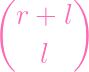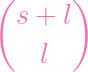(since order doesn't matter, this already covers the case of picking them in the reverse direction). Okay, phew, now Imma just go ahead and multiply by the (r + s)! and unwrap this baby (yes i'm assuming the dimension is r + s F U)

 (r + s)!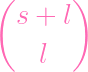= (r + s)!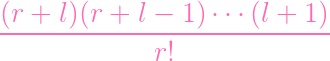⋅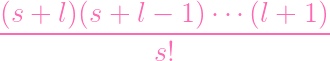OK: So, remember, we only care about the leading term (polynomial over l). The leading term in the numerator of the first fraction is lr, and the leading term in the numerator of the second fraction is ls. Hence, in total, the leading term is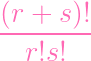lr+s

And that first term is indeed just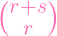=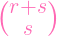, which matches our Queen's answer, and we are DUN.